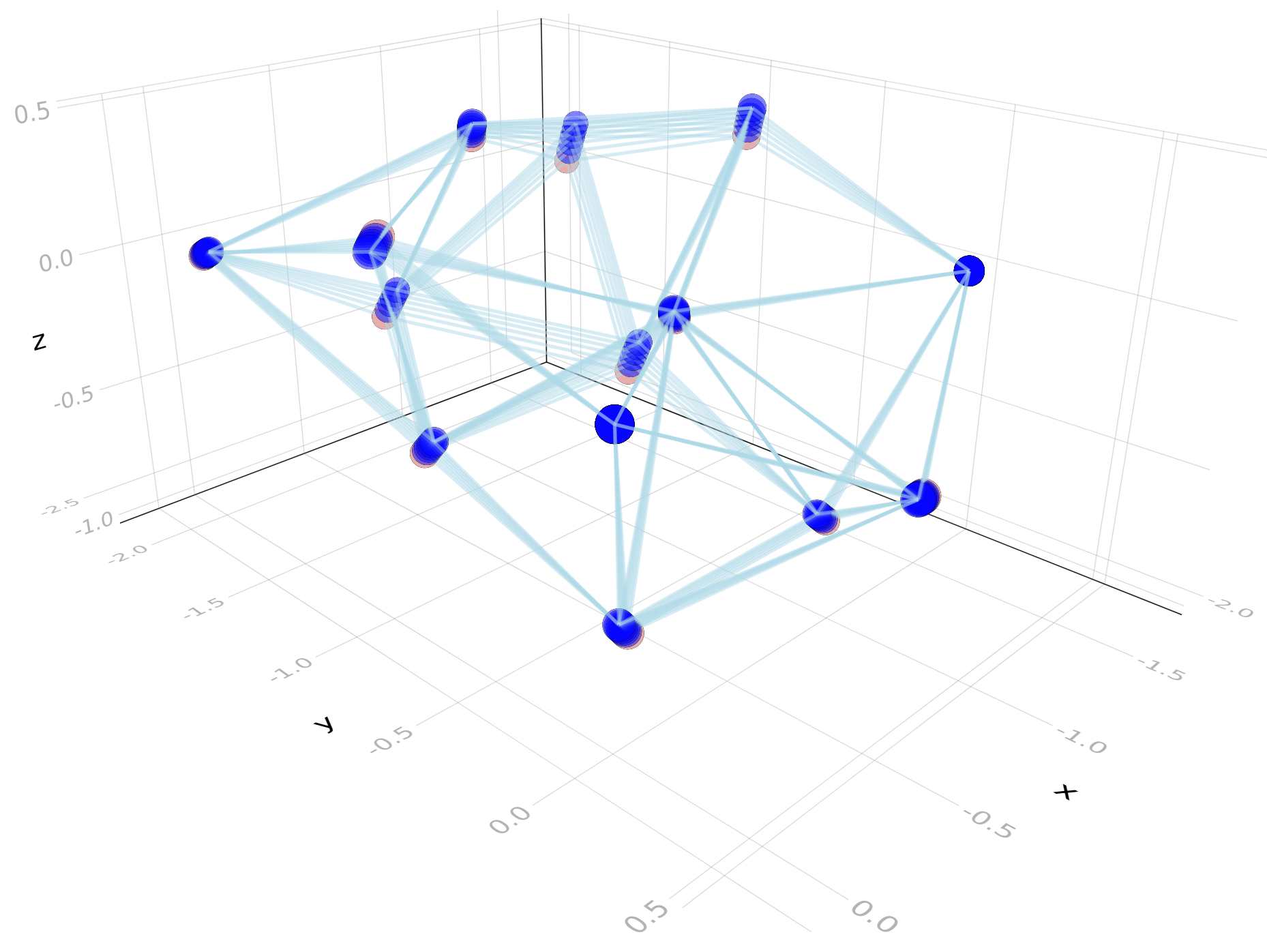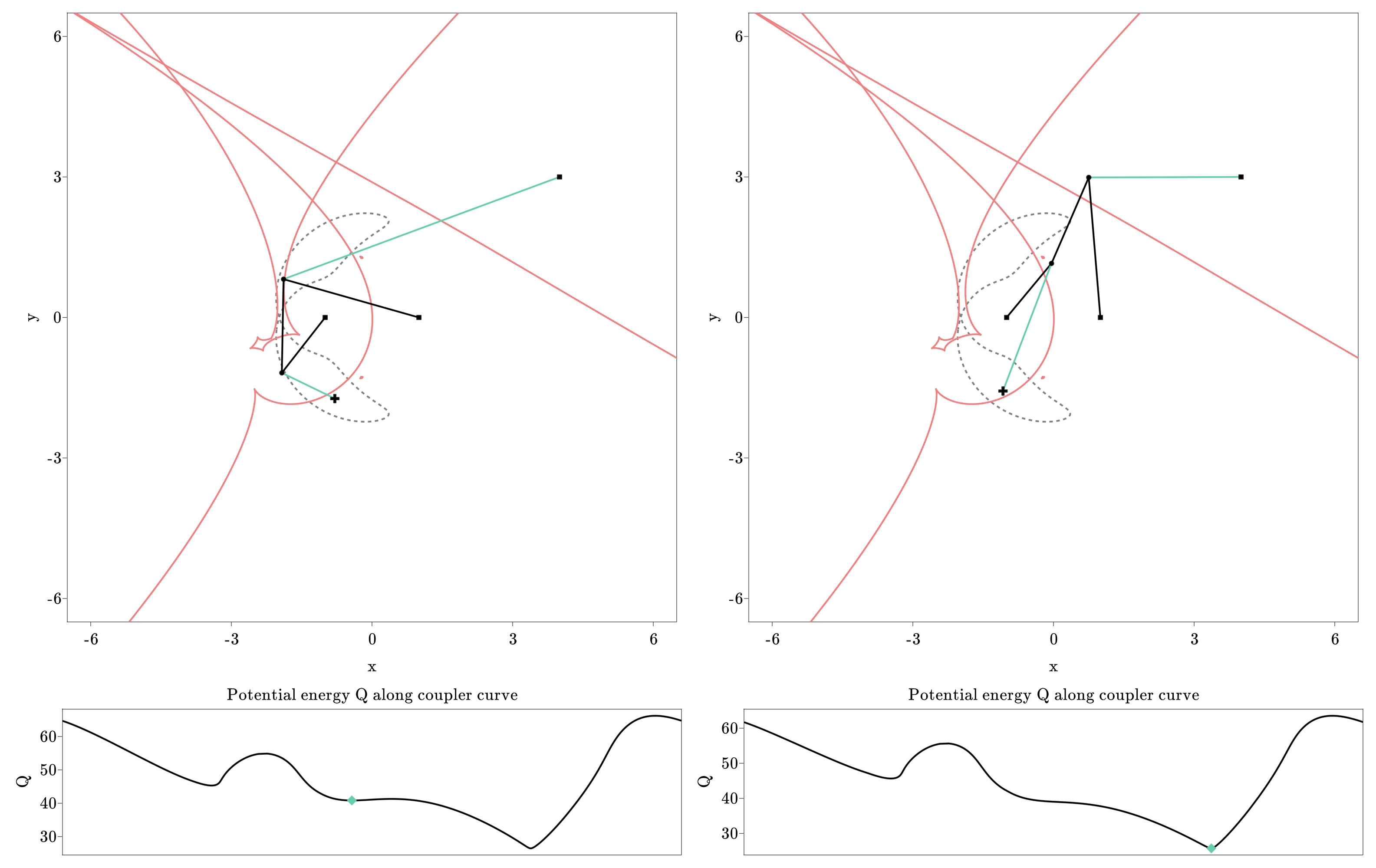# IN – Track A

## Applications of Algebra in the Geometry of Materials

Myfanwy Evans, Frank Lutz, Bernd Sturmfels

Project Members

Alexander Heaton (TU)

Project Duration

1 January 2020 – 31 December 2020

Located at

TU Berlin

### Description

We propose to develop novel algebraic geometry tools to analyze and understand the microstructure of materials. In particular, we intend to examine framework material structures and to develop the formalisation and fundamental concepts required to study these structures using computational algebra and real algebraic geometry. We begin with a family of instances generated using enumerative techniques from geometry and topology, and extend the available tools based on these case studies.

The overall goal of the project is the systematic analysis of framework microstructures from the perspective of real algebraic geometry. In particular, the concepts of periodicity of the structure and deformations of the structure will be rigorously defined and studied. We will study both framework materials as well as tensegrity materials, where the edge constraint equations are now inequalities. In frameworks, the edges are modelled as rigid bars that do not stretch or compress. However, we can view certain edges as cables, and allow them to stretch. For small perturbations the response should be linear according to Hooke’s law, and can be described by polynomial inequalities.

Practically, the study will be based around a particular set of basic material structures, namely vertices and edges connected in three-dimensional space. A given graph can be embedded into space in many ways. For each embedding, the mechanisms of the structure can be computed, but finding an embedding (or all embeddings) with specific properties is more difficult. The configuration space of these structures quickly becomes high-dimensional, and the configurations satisfying a specific property typically form a set with interesting geometry within this high-dimensional space. For example, configurations which admit nonnegative stresses on the edges that cancel at every vertex of the structure form an algebraic variety defined by (many) maximal minors of a rectangular incidence matrix. The emphasis is on the commonality of physical ideas of the structures alongside the algebraic varieties that parametrize the spatial realizations of the structure.

Project Webpages

Code for using semidefinite programming, computational algebra, and numerical algebraic geometry accompanying the first article below is available at the following webpage: https://github.com/alexheaton2/tensegrity. Code that accompanies the third article below can be found at the following webpage: https://github.com/saschatimme/Catastrophe.jl

Selected Publications

During 2020, work was completed on three research articles, which we describe below. First, the article Nonlinear algebra with tensegrity frameworks describes tools from nonlinear algebra which can be used in the study of bar-and-joint and tensegrity frameworks. Methods from computational algebra, algebraic geometry, semidefinite programming, and numerical algebraic geometry are employed. https://arxiv.org/abs/1908.08392.

Second, the article Epsilon local rigidity and numerical algebraic geometry (to appear in Journal of Algebra and its Applications) explores the idea that although it is often difficult to certify that a given configuration is an isolated point on the real algebraic set described by the edge constraints of a bar-and-joint framework, it is possible to decide whether the connected component of that point lies entirely within a sphere of small radius. In this case we conclude that if the framework can flex, it cannot flex too far. See https://arxiv.org/abs/2002.06154 and https://www.worldscientific.com/doi/abs/10.1142/S0219498822500098.

While bar frameworks are embedded graphs with edges viewed as rigid bars, tensegrity frameworks allow compression and tension elements. Typically, the rigidity community models tensegrity frameworks by edges that have inequality constraints on their lengths. However, a more realistic model is to treat the elastic elements using Hooke’s law. This leads to an energy minimization problem, with the geometry of stable equilibrium configurations arising as the solution to this optimization problem. In the article Catastrophe in Elastic Tensegrity Frameworks we show how methods from numerical algebraic geometry and optimization can be used to understand not only the equilibrium solutions, but the evolution of equilibrium solutions as certain control parameters vary in time. Catastrophe theory, also known as bifurcation theory, tries to understand when smooth changes in control parameters can cause discontinuous changes to the state of some system. In our article, we compute the algebraic catastrophe discriminants of elastic tensegrity frameworks using numerical algebraic geometry. In future work with biologists at Max Planck Institute of Colloids and Interfaces we will study the application of these methods to biofilms and mammalian tissues, which are known to retain internal tensional stresses. See https://arxiv.org/abs/2009.13408.

Selected Pictures##### (above) Flexes computed by parameter homotopy using numerical algebraic geometry
Because polynomials describe the condition that squared edge lengths remain constant, numerical algebraic geometry can be used to explore the configuration space of bar and joint frameworks, such as this framework arising from a sphere packing configuration.##### (above) Elastic four-bar framework before and after catastrophe.
Here we see the algebraic catastrophe discriminant of an elastic four-bar framework. Moving the control parameters across the catastrophe discriminant may trigger rapid, large-scale shape changes to the structure, as a local minimum disappears and the system is forced to rapidly evolve toward some new (far away) equilibrium configuration.

Please insert any kind of pictures (photos, diagramms, simulations, graphics) related to the project in the above right field (Image with Text), by choosing the green plus image on top of the text editor. (You will be directed to the media library where you can add new files.)
(We need pictures for a lot of purposes in different contexts, like posters, scientific reports, flyers, website,…
Please upload pictures that might be just nice to look at, illustrate, explain or summarize your work.)